# boxchart

Box chart (box plot)

Since R2020a

## Syntax

``boxchart(ydata)``
``boxchart(xgroupdata,ydata)``
``boxchart(___,'GroupByColor',cgroupdata)``
``boxchart(___,Name,Value)``
``boxchart(ax,___)``
``b = boxchart(___)``

## Description

example

````boxchart(ydata)` creates a box chart, or box plot, for each column of the matrix `ydata`. If `ydata` is a vector, then `boxchart` creates a single box chart.Each box chart displays the following information: the median, the lower and upper quartiles, any outliers (computed using the interquartile range), and the minimum and maximum values that are not outliers. For more information, see Box Chart (Box Plot).```

example

````boxchart(xgroupdata,ydata)` groups the data in the vector `ydata` according to the unique values in `xgroupdata` and plots each group of data as a separate box chart. `xgroupdata` determines the position of each box chart along the x-axis. `ydata` must be a vector, and `xgroupdata` must have the same length as `ydata`.```

example

````boxchart(___,'GroupByColor',cgroupdata)` uses color to differentiate between box charts. The software groups the data in the vector `ydata` according to the unique value combinations in `xgroupdata` (if specified) and `cgroupdata`, and plots each group of data as a separate box chart. The vector `cgroupdata` then determines the color of each box chart. `ydata` must be a vector, and `cgroupdata` must have the same length as `ydata`. Specify the `'GroupByColor'` name-value pair argument after any of the input argument combinations in the previous syntaxes.```

example

````boxchart(___,Name,Value)` specifies additional chart options using one or more name-value pair arguments. For example, you can compare sample medians using notches by specifying `'Notch','on'`. Specify the name-value pair arguments after all other input arguments. For a list of properties, see BoxChart Properties.```

example

````boxchart(ax,___)` plots into the axes specified by `ax` instead of into the current axes (`gca`). The argument `ax` can precede any of the input argument combinations in the previous syntaxes.```

example

````b = boxchart(___)` returns `BoxChart` objects. If you do not specify `cgroupdata`, then `b` contains one object. If you do specify it, then `b` contains a vector of objects, one for each unique value in `cgroupdata`. Use `b` to set properties of the box charts after creating them. For a list of properties, see BoxChart Properties.```

## Examples

collapse all

Create a single box chart from a vector of ages. Use the box chart to visualize the distribution of ages.

Load the `patients` data set. The `Age` variable contains the ages of 100 patients. Create a box chart to visualize the distribution of ages.

```load patients boxchart(Age) ylabel('Age (years)')```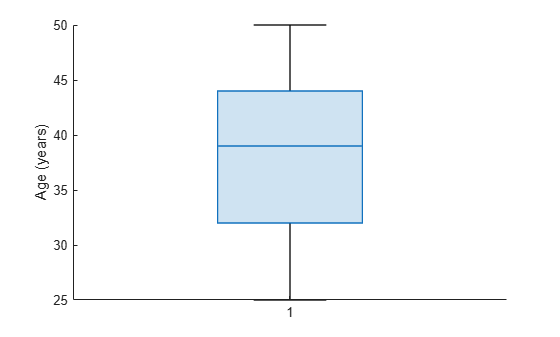The median patient age of 39 years is shown as the line inside the box. The lower and upper quartiles of 32 and 44 years are shown as the bottom and top edges of the box, respectively. The whiskers, or lines that extend below and above the box, have endpoints that correspond to the youngest and oldest patients. The youngest patient is 25 years old, and the oldest is 50 years old. The data set contains no outliers, which would be represented by small circles.

You can use data tips to get a summary of the data statistics. Hover over the box chart to see the data tip.

Use box charts to compare the distribution of values along the columns and the rows of a magic square.

Create a magic square with 10 rows and 10 columns.

`Y = magic(10)`
```Y = 10×10 92 99 1 8 15 67 74 51 58 40 98 80 7 14 16 73 55 57 64 41 4 81 88 20 22 54 56 63 70 47 85 87 19 21 3 60 62 69 71 28 86 93 25 2 9 61 68 75 52 34 17 24 76 83 90 42 49 26 33 65 23 5 82 89 91 48 30 32 39 66 79 6 13 95 97 29 31 38 45 72 10 12 94 96 78 35 37 44 46 53 11 18 100 77 84 36 43 50 27 59 ```

Create a box chart for each column of the magic square. Each column has a similar median value (around `50`). However, the first five columns of `Y` have greater interquartile ranges than the last five columns of `Y`. The interquartile range is the distance between the upper quartile (top edge of the box) and the lower quartile (bottom edge of the box).

```boxchart(Y) xlabel('Column') ylabel('Value')```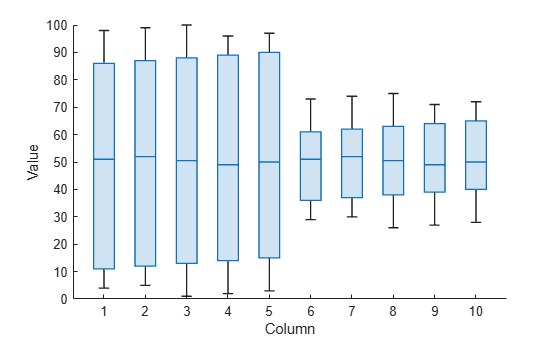Create a box chart for each row of the magic square. Each row has a similar interquartile range, but the median values differ across the rows.

```boxchart(Y') xlabel('Row') ylabel('Value')```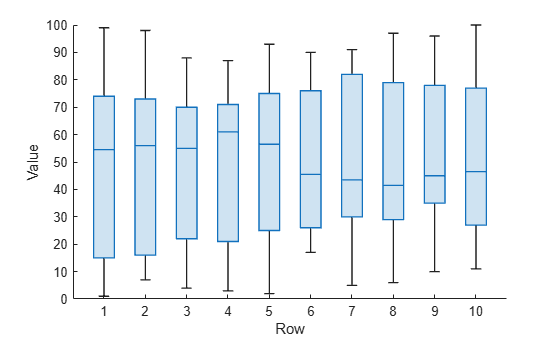Plot the magnitudes of earthquakes according to the month in which they occurred. Use a vector of earthquake magnitudes and a grouping variable indicating the month of each earthquake. For each group of data, create a box chart and place it in the specified position along the x-axis.

Read a set of tsunami data into the workspace as a table. The data set includes information on earthquakes as well as other causes of tsunamis. Display the first eight rows, showing the month, cause, and earthquake magnitude columns of the table.

```tsunamis = readtable('tsunamis.xlsx'); tsunamis(1:8,["Month","Cause","EarthquakeMagnitude"])```
```ans=8×3 table Month Cause EarthquakeMagnitude _____ __________________ ___________________ 10 {'Earthquake' } 7.6 8 {'Earthquake' } 6.9 12 {'Volcano' } NaN 3 {'Earthquake' } 8.1 3 {'Earthquake' } 4.5 5 {'Meteorological'} NaN 11 {'Earthquake' } 9 3 {'Earthquake' } 5.8 ```

Create the table `earthquakes`, which contains data for the tsunamis caused by earthquakes.

`unique(tsunamis.Cause)`
```ans = 8x1 cell {0x0 char } {'Earthquake' } {'Earthquake and Landslide'} {'Landslide' } {'Meteorological' } {'Unknown Cause' } {'Volcano' } {'Volcano and Landslide' } ```
```idx = contains(tsunamis.Cause,'Earthquake'); earthquakes = tsunamis(idx,:);```

Group the earthquake magnitudes based on the month in which the corresponding tsunamis occurred. For each month, display a separate box chart. For example, `boxchart` uses the fourth, fifth, and eighth earthquake magnitudes, as well as others, to create the third box chart, which corresponds to the third month.

```boxchart(earthquakes.Month,earthquakes.EarthquakeMagnitude) xlabel('Month') ylabel('Earthquake Magnitude')```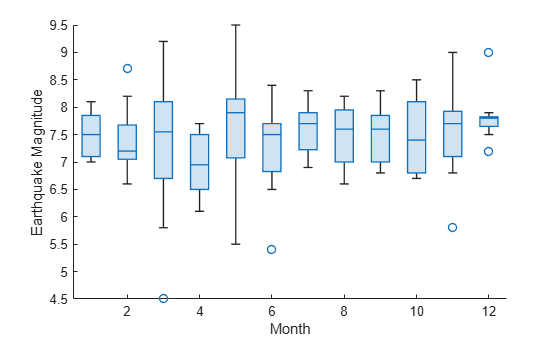Notice that because the month values are numeric, the x-axis ruler is also numeric.

For more descriptive month names, convert the `earthquakes.Month` column to a `categorical` variable.

```monthOrder = ["Jan","Feb","Mar","Apr","May","Jun","Jul", ... "Aug","Sep","Oct","Nov","Dec"]; namedMonths = categorical(earthquakes.Month,1:12,monthOrder);```

Create the same box charts as before, but use the `categorical` variable `namedMonths` instead of the numeric month values. The x-axis ruler is now categorical, and the order of the categories in `namedMonths` determines the order of the box charts.

```boxchart(namedMonths,earthquakes.EarthquakeMagnitude) xlabel('Month') ylabel('Earthquake Magnitude')```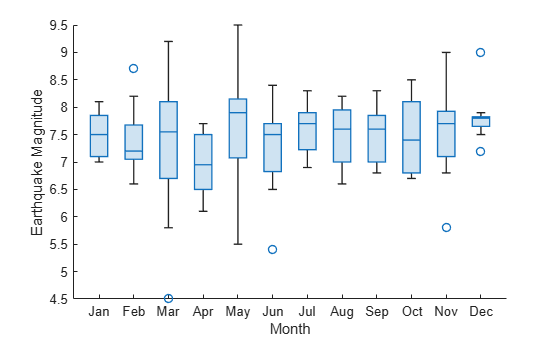Group medical patients based on their age, and for each age group, create a box chart of diastolic blood pressure values.

Load the `patients` data set. The `Age` and `Diastolic` variables contain the ages and diastolic blood pressure levels of 100 patients.

`load patients`

Group the patients into five age bins. Find the minimum and maximum ages, and then divide the range between them into five-year bins. Bin the values in the `Age` variable by using the `discretize` function. Use the bin names in `bins`. The resulting `groupAge` variable is a `categorical` variable.

`min(Age)`
```ans = 25 ```
`max(Age)`
```ans = 50 ```
```binEdges = 25:5:50; bins = {'late 20s','early 30s','late 30s','early 40s','late 40s+'}; groupAge = discretize(Age,binEdges,'categorical',bins);```

Create a box chart for each age group. Each box chart shows the diastolic blood pressure values of the patients in that group.

```boxchart(groupAge,Diastolic) xlabel('Age Group') ylabel('Diastolic Blood Pressure')```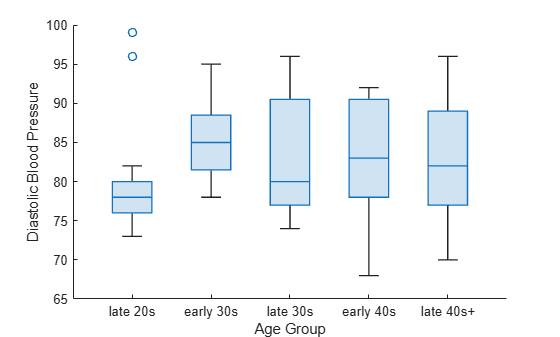Use two grouping variables to group data and to position and color the resulting box charts.

Load the sample file `TemperatureData.csv`, which contains average daily temperatures from January 2015 through July 2016. Read the file into a table.

`tbl = readtable('TemperatureData.csv');`

Convert the `tbl.Month` variable to a `categorical` variable. Specify the order of the categories.

```monthOrder = {'January','February','March','April','May','June','July', ... 'August','September','October','November','December'}; tbl.Month = categorical(tbl.Month,monthOrder);```

Create box charts showing the distribution of temperatures during each month of each year. Specify `tbl.Month` as the positional grouping variable. Specify `tbl.Year` as the color grouping variable by using the `'GroupByColor'` name-value pair argument. Notice that `tbl` does not contain data for some months of 2016.

```boxchart(tbl.Month,tbl.TemperatureF,'GroupByColor',tbl.Year) ylabel('Temperature (F)') legend```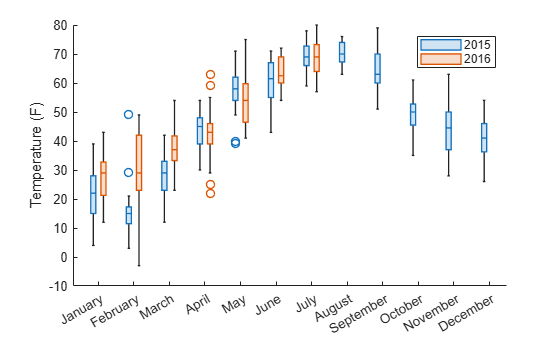In this figure, you can easily compare the distribution of temperatures for one particular month across multiple years. For example, you can see that February temperatures varied much more in 2016 than in 2015.

Create box charts, and plot the mean values over the box charts by using `hold on`.

Load the `patients` data set. Convert `SelfAssessedHealthStatus` to an ordinal `categorical` variable because the categories `Poor`, `Fair`, `Good`, and `Excellent` have a natural order.

```load patients healthOrder = {'Poor','Fair','Good','Excellent'}; SelfAssessedHealthStatus = categorical(SelfAssessedHealthStatus, ... healthOrder,'Ordinal',true);```

Group the patients according to their self-assessed health status, and find the mean patient weight for each group.

`meanWeight = groupsummary(Weight,SelfAssessedHealthStatus,'mean');`

Compare the weights for each group of patients by using box charts. Plot the mean weights over the box charts.

```boxchart(SelfAssessedHealthStatus,Weight) hold on plot(meanWeight,'-o') hold off legend(["Weight Data","Weight Mean"])```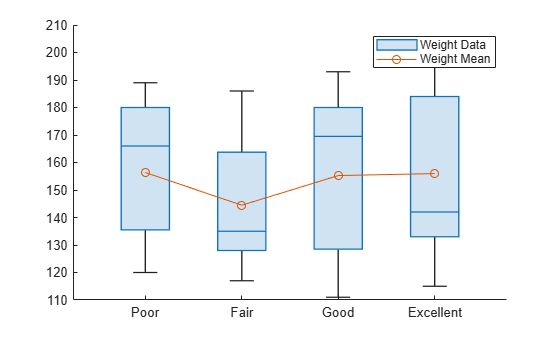Use notches to determine whether median values are significantly different from each other.

Load the `patients` data set. Split the patients according to their location. For each group of patients, create a box chart of their weights. Specify `'Notch','on'` so that each box includes a tapered, shaded region called a notch. Box charts whose notches do not overlap have different medians at the 5% significance level.

```load patients boxchart(categorical(Location),Weight,'Notch','on') ylabel('Weight (lbs)')```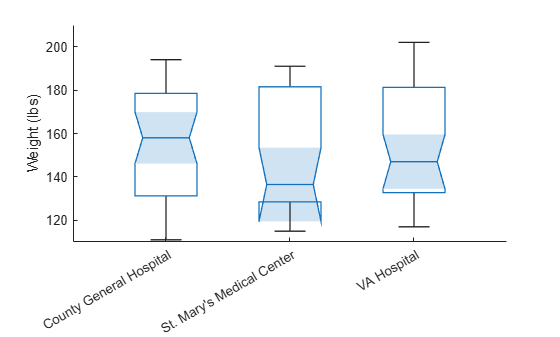In this example, the three notches overlap, showing that the three weight medians are not significantly different.

Display a side-by-side pair of box charts using the `tiledlayout` and `nexttile` functions.

Load the `patients` data set. Convert `Smoker` to a `categorical` variable with the descriptive category names `Smoker` and `Nonsmoker` rather than `1` and `0`.

```load patients Smoker = categorical(Smoker,logical([1 0]),{'Smoker','Nonsmoker'});```

Create a 1-by-2 tiled chart layout using the `tiledlayout` function. Create the first set of axes `ax1` within it by calling the `nexttile` function. In the first set of axes, display two box charts of systolic blood pressure values, one for smokers and the other for nonsmokers. Create the second set of axes `ax2` within the tiled chart layout by calling the `nexttile` function. In the second set of axes, do the same for diastolic blood pressure.

```tiledlayout(1,2) % Left axes ax1 = nexttile; boxchart(ax1,Systolic,'GroupByColor',Smoker) ylabel(ax1,'Systolic Blood Pressure') legend % Right axes ax2 = nexttile; boxchart(ax2,Diastolic,'GroupByColor',Smoker) ylabel(ax2,'Diastolic Blood Pressure') legend```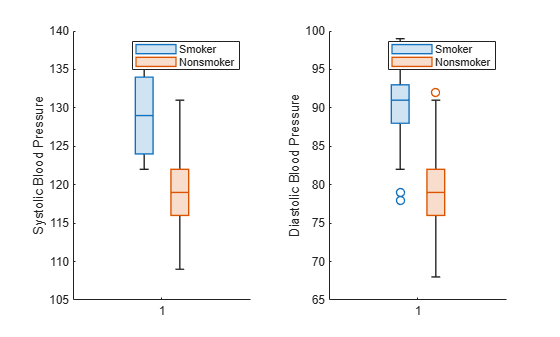Create a set of color-coded box charts, returned as a vector of `BoxChart` objects. Use the vector to change the color of one box chart.

Load the `patients` data set. Convert `Gender` and `Smoker` to `categorical` variables. Specify the descriptive category names `Smoker` and `Nonsmoker` rather than `1` and `0`.

```load patients Gender = categorical(Gender); Smoker = categorical(Smoker,logical([1 0]),{'Smoker','Nonsmoker'});```

Combine the `Gender` and `Smoker` variables into one grouping variable `cgroupdata`. Create box charts showing the distribution of diastolic blood pressure levels for each pairing of gender and smoking status. `b` is a vector of `BoxChart` objects, one for each group of data.

```cgroupdata = Gender.*Smoker; b = boxchart(Diastolic,'GroupByColor',cgroupdata)```
```b = 4x1 BoxChart array: BoxChart BoxChart BoxChart BoxChart ```
`legend('Location','southeast')`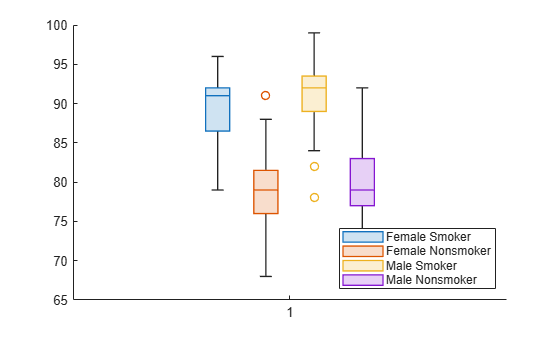Update the color of the third box chart by using the `SeriesIndex` property. Updating the `SeriesIndex` property changes both the box face color and the outlier marker color.

`b(3).SeriesIndex = 6;`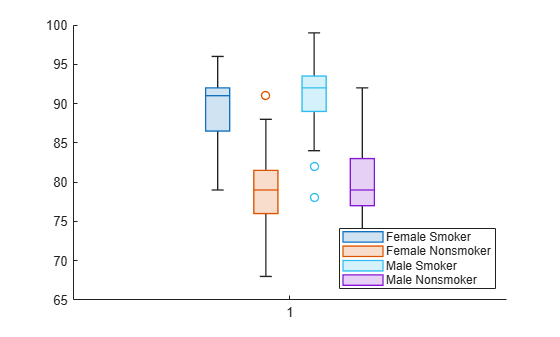Create a box chart from power outage data with many outliers, and make it easier to distinguish them visually by changing the properties of the `BoxChart` object. Find the indices for the outlier entries.

Read power outage data into the workspace as a table. Display the first few rows of the table.

```outages = readtable('outages.csv'); head(outages)```
``` Region OutageTime Loss Customers RestorationTime Cause _____________ ________________ ______ __________ ________________ ___________________ {'SouthWest'} 2002-02-01 12:18 458.98 1.8202e+06 2002-02-07 16:50 {'winter storm' } {'SouthEast'} 2003-01-23 00:49 530.14 2.1204e+05 NaT {'winter storm' } {'SouthEast'} 2003-02-07 21:15 289.4 1.4294e+05 2003-02-17 08:14 {'winter storm' } {'West' } 2004-04-06 05:44 434.81 3.4037e+05 2004-04-06 06:10 {'equipment fault'} {'MidWest' } 2002-03-16 06:18 186.44 2.1275e+05 2002-03-18 23:23 {'severe storm' } {'West' } 2003-06-18 02:49 0 0 2003-06-18 10:54 {'attack' } {'West' } 2004-06-20 14:39 231.29 NaN 2004-06-20 19:16 {'equipment fault'} {'West' } 2002-06-06 19:28 311.86 NaN 2002-06-07 00:51 {'equipment fault'} ```

Create a `BoxChart` object `b` from the `outages.Customers` values, which indicate how many customers were affected by each power outage. `boxchart` discards entries with `NaN` values.

```b = boxchart(outages.Customers); ylabel('Number of Customers')```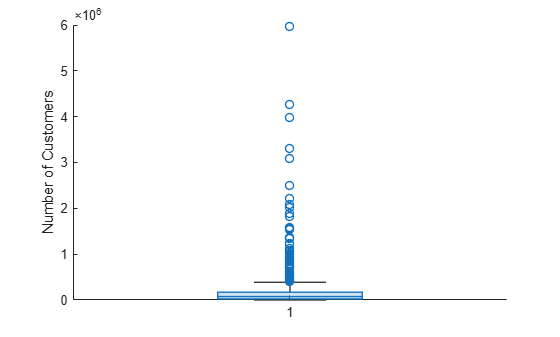The plot contains many outliers. To better see them, jitter the outliers and change the outlier marker style. When you set the `JitterOutliers` property of the `BoxChart` object to `'on'`, the software randomly displaces the outlier markers horizontally so that they are unlikely to overlap perfectly. The values and vertical positions of the outliers are unchanged.

```b.JitterOutliers = 'on'; b.MarkerStyle = '.';```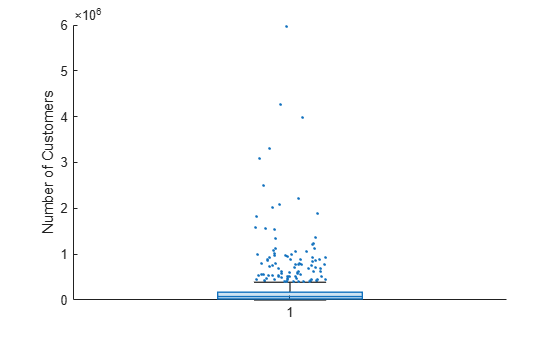You can now more easily see the distribution of outliers.

To find the outlier indices, use the `isoutlier` function. Specify the `'quartiles'` method of computing outliers to match the `boxchart` outlier definition. Use the indices to create the `outliers` table, which contains a subset of the `outages` data. Notice that `isoutlier` identifies 96 outliers.

```idx = isoutlier(outages.Customers,'quartiles'); outliers = outages(idx,:); size(outliers,1)```
```ans = 96 ```

Because of all the outliers, the quartiles of the box chart are hard to see. To inspect them, change the y-axis limits.

`ylim([0 4e5])`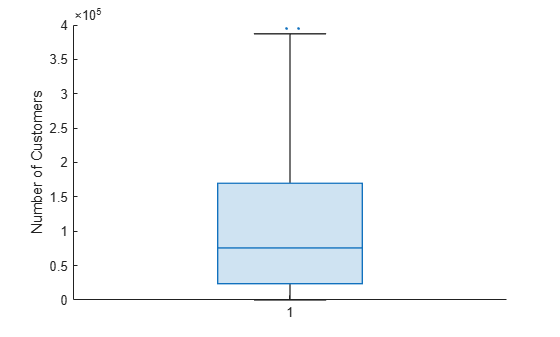## Input Arguments

collapse all

Sample data, specified as a numeric vector or matrix.

• If `ydata` is a matrix, then `boxchart` creates a box chart for each column of `ydata`.

• If `ydata` is a vector and you do not specify `xgroupdata` or `cgroupdata`, then `boxchart` creates a single box chart.

• If `ydata` is a vector and you do specify `xgroupdata` or `cgroupdata`, then `boxchart` creates a box chart for each unique value combination in `xgroupdata` and `cgroupdata`.

Data Types: `single` | `double` | `int8` | `int16` | `int32` | `int64` | `uint8` | `uint16` | `uint32` | `uint64`

Positional grouping variable, specified as a numeric or categorical vector. `xgroupdata` must have the same length as the vector `ydata`; you cannot specify `xgroupdata` when `ydata` is a matrix.

`boxchart` groups the data in `ydata` according to the unique value combinations in `xgroupdata` and `cgroupdata`. The function creates a box chart for each group of data and positions each box chart at the corresponding `xgroupdata` value. By default, `boxchart` vertically orients the box charts and displays the `xgroupdata` values along the x-axis. You can change the box chart orientation by using the `Orientation` property.

Data Types: `single` | `double` | `int8` | `int16` | `int32` | `int64` | `uint8` | `uint16` | `uint32` | `uint64` | `categorical`

Color grouping variable, specified as a numeric vector, categorical vector, logical vector, string array, character vector, or cell array of character vectors. `cgroupdata` must have the same length as the vector `ydata`; you cannot specify `cgroupdata` when `ydata` is a matrix.

`boxchart` groups the data in `ydata` according to the unique value combinations in `xgroupdata` and `cgroupdata`. The function creates a box chart for each group of data and assigns the same color to groups with the same `cgroupdata` value.

Data Types: `single` | `double` | `int8` | `int16` | `int32` | `int64` | `uint8` | `uint16` | `uint32` | `uint64` | `categorical` | `logical` | `string` | `char` | `cell`

Target axes, specified as an `Axes` object. If you do not specify the axes, then `boxchart` uses the current axes (`gca`).

### Name-Value Arguments

Specify optional pairs of arguments as `Name1=Value1,...,NameN=ValueN`, where `Name` is the argument name and `Value` is the corresponding value. Name-value arguments must appear after other arguments, but the order of the pairs does not matter.

Before R2021a, use commas to separate each name and value, and enclose `Name` in quotes.

Example: ```boxchart([rand(10,4); 4*rand(1,4)],'BoxFaceColor',[0 0.5 0],'MarkerColor',[0 0.5 0])``` creates box charts with green boxes and green outliers, if applicable.

The `BoxChart` properties listed here are only a subset. For a complete list, see BoxChart Properties.

Box color, specified as an RGB triplet, hexadecimal color code, color name, or short name. The box includes the box edges and median line. To specify the color of the box edges and median line separately, you can use the `BoxEdgeColor` property. To specify the color of the median line only, use the `BoxMedianLineColor` property.

For a custom color, specify an RGB triplet or a hexadecimal color code.

• An RGB triplet is a three-element row vector whose elements specify the intensities of the red, green, and blue components of the color. The intensities must be in the range `[0,1]`, for example, ```[0.4 0.6 0.7]```.

• A hexadecimal color code is a string scalar or character vector that starts with a hash symbol (`#`) followed by three or six hexadecimal digits, which can range from `0` to `F`. The values are not case sensitive. Therefore, the color codes `"#FF8800"`, `"#ff8800"`, `"#F80"`, and `"#f80"` are equivalent.

Alternatively, you can specify some common colors by name. This table lists the named color options, the equivalent RGB triplets, and hexadecimal color codes.

Color NameShort NameRGB TripletHexadecimal Color CodeAppearance
`"red"``"r"``[1 0 0]``"#FF0000"``"green"``"g"``[0 1 0]``"#00FF00"``"blue"``"b"``[0 0 1]``"#0000FF"``"cyan"` `"c"``[0 1 1]``"#00FFFF"``"magenta"``"m"``[1 0 1]``"#FF00FF"``"yellow"``"y"``[1 1 0]``"#FFFF00"``"black"``"k"``[0 0 0]``"#000000"``"white"``"w"``[1 1 1]``"#FFFFFF"``"none"`Not applicableNot applicableNot applicableNo color

Here are the RGB triplets and hexadecimal color codes for the default colors MATLAB® uses in many types of plots.

`[0 0.4470 0.7410]``"#0072BD"``[0.8500 0.3250 0.0980]``"#D95319"``[0.9290 0.6940 0.1250]``"#EDB120"``[0.4940 0.1840 0.5560]``"#7E2F8E"``[0.4660 0.6740 0.1880]``"#77AC30"``[0.3010 0.7450 0.9330]``"#4DBEEE"``[0.6350 0.0780 0.1840]``"#A2142F"`Example: ```b = boxchart(rand(10,1),'BoxFaceColor','red')```

Example: `b.BoxFaceColor = [0 0.5 0.5];`

Example: `b.BoxFaceColor = '#EDB120';`

Outlier style, specified as one of the options listed in this table.

MarkerDescriptionResulting Marker
`"o"`Circle`"+"`Plus sign`"*"`Asterisk`"."`Point`"x"`Cross`"_"`Horizontal line`"|"`Vertical line`"square"`Square`"diamond"`Diamond`"^"`Upward-pointing triangle`"v"`Downward-pointing triangle`">"`Right-pointing triangle`"<"`Left-pointing triangle`"pentagram"`Pentagram`"hexagram"`Hexagram`"none"`No markersNot applicable

Example: `b = boxchart([rand(10,1);2],'MarkerStyle','x')`

Example: `b.MarkerStyle = 'x';`

Outlier marker displacement, specified as `'on'` or `'off'`, or as numeric or logical `1` (`true`) or `0` (`false`). A value of `'on'` is equivalent to `true`, and `'off'` is equivalent to `false`. Thus, you can use the value of this property as a logical value. The value is stored as an on/off logical value of type `matlab.lang.OnOffSwitchState`.

If you set the `JitterOutliers` property to `'on'`, then `boxchart` randomly displaces the outlier markers along the `XData` direction to help you distinguish between outliers that have similar `ydata` values. For an example, see Visualize and Find Outliers.

Example: `b = boxchart([rand(20,1);2;2;2],'JitterOutliers','on')`

Example: `b.JitterOutliers = 'on';`

Median comparison display, specified as `'on'` or `'off'`, or as numeric or logical `1` (`true`) or `0` (`false`). A value of `'on'` is equivalent to `true`, and `'off'` is equivalent to `false`. Thus, you can use the value of this property as a logical value. The value is stored as an on/off logical value of type `matlab.lang.OnOffSwitchState`.

If you set the `Notch` property to `'on'`, then `boxchart` creates a tapered, shaded region around each median. Box charts whose notches do not overlap have different medians at the 5% significance level. For more information, see Box Chart (Box Plot).

Notches can extend beyond the lower and upper quartiles.

Example: `b = boxchart(rand(10,2),'Notch','on')`

Example: `b.Notch = 'on';`

Orientation of box charts, specified as `'vertical'` or `'horizontal'`. By default, the box charts are vertically orientated, so that the `ydata` statistics are aligned with the y-axis. Regardless of the orientation, `boxchart` stores the `ydata` values in the `YData` property of the `BoxChart` object.

Example: `b = boxchart(rand(10,1),'Orientation','horizontal')`

Example: `b.Orientation = 'horizontal';`

## Output Arguments

collapse all

Box charts, returned as a vector of `BoxChart` objects. `b` contains one `BoxChart` object for each unique value in `cgroupdata`. For more information, see BoxChart Properties.

collapse all

### Box Chart (Box Plot)

A box chart, or box plot, provides a visual representation of summary statistics for a data sample. Given numeric data, the corresponding box chart displays the following information: the median, the lower and upper quartiles, any outliers (computed using the interquartile range), and the minimum and maximum values that are not outliers.

• The line inside of each box is the sample median. You can compute the value of the median using the `median` function.

• The top and bottom edges of each box are the upper and lower quartiles, respectively. The distance between the top and bottom edges is the interquartile range (IQR).

For more information on how the quartiles are computed, see `quantile`, where the upper quartile corresponds to the 0.75 quantile and the lower quartile corresponds to the 0.25 quantile.

• Outliers are values that are more than 1.5 · IQR away from the top or bottom of the box. By default, `boxchart` displays each outlier using an `'o'` symbol. The outlier computation is comparable to that of the `isoutlier` function with the `'quartiles'` method.

• The whiskers are lines that extend above and below each box. One whisker connects the upper quartile to the nonoutlier maximum (the maximum data value that is not an outlier), and the other connects the lower quartile to the nonoutlier minimum (the minimum data value that is not an outlier).

• Notches help you compare sample medians across multiple box charts. When you specify `'Notch','on'`, the `boxchart` function creates a tapered, shaded region around each median. Box charts whose notches do not overlap have different medians at the 5% significance level. The significance level is based on a normal distribution assumption, but the median comparison is reasonably robust for other distributions.

The top and bottom edges of the notch region correspond to $m+\left(1.57\cdot IQR\right)/\sqrt{n}$ and $m-\left(1.57\cdot IQR\right)/\sqrt{n}$, respectively, where m is the median, IQR is the interquartile range, and n is the number of data points, excluding `NaN` values.## Tips

• Use data tips to explore the data in `BoxChart` objects. Some options are not available in the Live Editor.

• You can add two types of data tips to a `BoxChart` object: one for each box chart and one for each outlier. A general data tip appears at the nonoutlier maximum value, regardless of where you click on the box chart.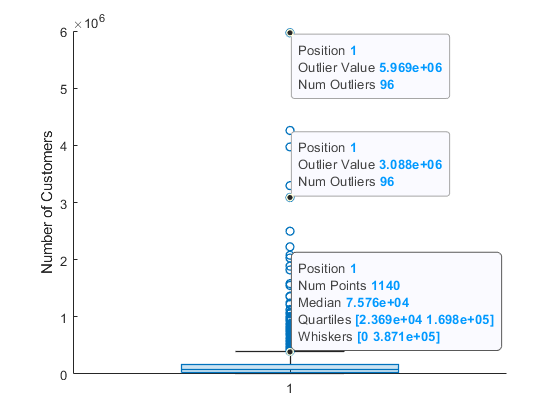Note

The displayed `Num Points` value includes `NaN` values in the corresponding `ydata`, but `boxchart` discards the `NaN` values before computing the box chart statistics.

• You can use the `datatip` function to add more data tips to a `BoxChart` object, but the indexing of data tips differs from other charts. `boxchart` first assigns indices to the box charts and then assigns indices to the outliers. For example, if a `BoxChart` object `b` displays two box charts and one outlier, `datatip(b,'DataIndex',3)` creates a data tip at the outlier point.

## Version History

Introduced in R2020a